## Thursday, 17 January 2013

### Addition of Two Numbers Java Programming Code

Java program to add two numbers

#### Simple Program to Add two numbers in Java

//A Simple Program to Add Two Numbers in Java Program.

class AddTwoNumbers        //Class Declaration
{
public static void main(String args[]) //Main Function
{
int x, y, z;      // Variable Declaration
x = 10;           //Assigning 10 to the variable x.
y = 20;           //Assigning 20 to the variable y.
z = x + y;        //Expression to Add two variables x, y and save the result to z
System.out.println("Sum of x and y = "+z); //This line output the value of z on the Screen
}
}

### Output:

Sum of x and y =30

#### 42 comments :

1.y = 20; //Assigning 10 to the variable y.
Place of Above Line
y = 20; //Assigning 20 to the variable y

2.y = 20; //Assigning 20 to the variable y
place of above line

1.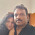Thanks and updated..

3.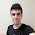4.thanks, learn much more

5.This comment has been removed by a blog administrator.

6.I AM FROM ANONYMOUS NOOB CODES

7.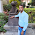but i want take two numbers by user,
how to take numbers by user and then calculate it's sum
please tell me sir
thanks.

1.2.import java.io.*;
class sid
{
public static void main(String [] args)throws IOException
{
System.out.println("Enter two no.");
int z=x*y;
int a=x+y;
System.out.println("Siddhant"+z+a);
}
}

//Sorry cant explain how it is but this is is try it

3.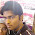i think it need scanner

4.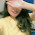import java.util.Scanner;
public class Addition {

public static void main(String[] args) {
// TODO Auto-generated method stub

int a,b,c;
System.out.println("Enter the two numbers here");

Scanner me = new Scanner(System.in);

a= me.nextInt();
b=me.nextInt();
c=a+b;
System.out.println("Addition of two values= " +c);

}

}

[this one is simpler and easy]

8.Post is good for absolute noobs. Check this out : Short article on how to learn java programming

9.Post is good for absolute noobs. Check this out : Short article on how to learn java programming Java programming tutorial

10.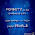Instead of buffer readers u can also take scanner class.

11.import java.util.Scanner;

{
public static void main(String args[])
{
int x, y, z;
System.out.println("Enter two integers to calculate their sum ");
Scanner in = new Scanner(System.in);
x = in.nextInt();
y = in.nextInt();
z = x + y;
System.out.println("Sum of entered integers = "+z);
}
}

1.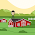im running this on Mac im getting 11 errors

12.13.Post is good..
How about printing like this :

int x=10,y=20;
System.out.println("sum : "+(x+y));

See this as well : Best way to learn java programming

14.So helpful. Thanks for sharing.
IELTS classes in Kuwait

15.how short datatype use in addition method

16.how short datatype use in addition method

17.18.sir ,,,can u tell me how to run java program using cmd

1.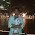first
javac class name.java

then
java class name

2.This comment has been removed by the author.

3.kindly visit the link: http://java191.blogspot.com.ng/2017/02/how-to-compile-and-execute-java-program.html

19.import java.util.Scanner;

{
public static void main(String args[])
{
int x, y, z;
System.out.println("Enter two integers to calculate their sum ");
Scanner in = new Scanner(System.in);
x = in.nextInt();
y = in.nextInt();
z = x + y;
System.out.println("Sum of entered integers = "+z);
}
}

1.hi, im running this same code on mac and I am getting 11 errors

20.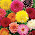if i want to declare variables with in class then how to write a program for that

1.You can just add public static int x, y, z; in the class to use the variables. Java program to add two numbers

21.if i want to declare variables with in class then how to write a program for that

22.Check here with the steps : Java program to add two numbers

23.Write A Programs
Good Explanation.....

24.in addition to the program. We can modify the program in such a way that the user input two numbers then it displays the sum of the two numbers in a message dialog box. You can check out the program and detailed explanation of the code through the following link: http://java191.blogspot.com.ng/2017/02/java-addition-program-that-uses.html

25.Good and nice information, thanks for sharing your views and ideas...
Web Designing Training in chennai | PHP Training in chennai

26.Great post aboutjava programs
thanks

27.Data type not mention....It generate error

28.In mahrien fariha's method

29.without using scanner and we will not declare the variables in the program it can possiable

30.Please tell about Return type with example

31.Since all execution happens on the program, Javascript is in charge of the majority of the intelligence on a website page. code online easily